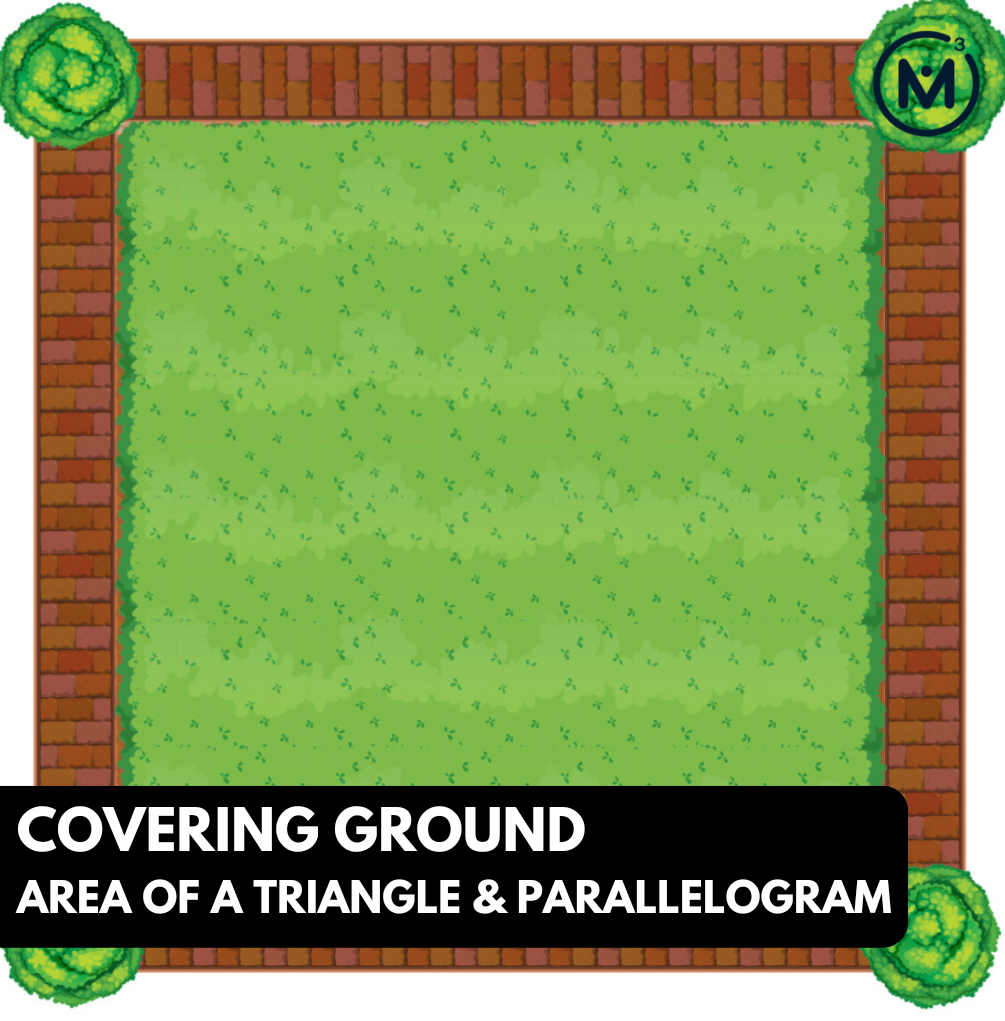## COVERING GROUND [DAY 5]

### AREA OF A TRIANGLE & PARALLELOGRAM

Investigate the area of a triangle and area of a parallelogram to generalize a formula for each.

## Intentionality & Unit Overview### Access each lesson from this unit using the navigation links below

Students will demonstrate the concepts and skills that they have developed through these contexts and learning experiences.

## Intentionality…

The purpose of this assessment is for students to demonstrate their understanding of the following big ideas.

• The area of a rectangle can be determined by multiplying the length of its base by the length of its height;
• The area of a rectangle is used to determine the area formulas for other polygons;
• Using “base” and “height” rather than “length” and “width” can support student understanding when determining the area formulas for triangles and all quadrilaterals;
• A parallelogram is any quadrilateral with two sets of parallel sides;
• A rectangle is a parallelogram;
• Any triangle can be doubled to create a parallelogram;
• Congruent shapes have the same size and shape, but not necessarily the same orientation;
• Any parallelogram can be decomposed and recomposed to build a rectangle with the same area.

The independent assessment will allow students to demonstrate concepts and skills related to the following learning goals:

• I will calculate the area of a triangle;
• I will calculate the area of parallelogram;
• I will determine the dimensions of a triangle and a parallelogram when given the area; and,
• I will demonstrate an understanding of the relationship between the area of a rectangle, and the area of both triangles and parallelograms.

Students might write and/or model their task independently. Students can also be interviewed by their teacher.

An alternative assessment opportunity should be offered if this tool will not successfully capture what the student knows, understands or can do.

## Math Talk

Login/Join to access the entire Teacher Guide, downloadable slide decks and printable handouts for this lesson and all problem based units.

## Visual Math Talk Prompt #1

Login/Join to access the entire Teacher Guide, downloadable slide decks and printable handouts for this lesson and all problem based units.

## Visual Math Talk Prompt #2

Login/Join to access the entire Teacher Guide, downloadable slide decks and printable handouts for this lesson and all problem based units.

## Visual Math Talk Prompt #3

Login/Join to access the entire Teacher Guide, downloadable slide decks and printable handouts for this lesson and all problem based units.

## Visual Math Talk Prompt #4

Login/Join to access the entire Teacher Guide, downloadable slide decks and printable handouts for this lesson and all problem based units.

## Assessment

Login/Join to access the entire Teacher Guide, downloadable slide decks and printable handouts for this lesson and all problem based units.

## Assessment: Area of a Triangles and Parallelograms

Login/Join to access the entire Teacher Guide, downloadable slide decks and printable handouts for this lesson and all problem based units.

## Considerations to Promote Student Growth

Login/Join to access the entire Teacher Guide, downloadable slide decks and printable handouts for this lesson and all problem based units.

Login/Join to access the entire Teacher Guide, downloadable slide decks and printable handouts for this lesson and all problem based units.

## Educator Discussion Area

Login/Join to access the entire Teacher Guide, downloadable slide decks and printable handouts for this lesson and all problem based units.

## Explore Our 60+ Problem Based Units

This Make Math Moments Lesson was designed to spark curiosity for a multi-day unit of study with built in purposeful practice, number talks and extensions to elicit and emerge strategies and mathematical models.

Dig into our other units of study and view by concept continuum, grade or topic!